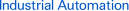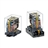Page top# Item list of MM

Power Relay

###Power Relay MM

Stable Contact Reliability and Long Life

about this Product Family

Displaying individual registered product index. Select and click for details.

Count

All Items 1 - 17 of 17

Model NumberDescription
Standard type, Single, Plug-in terminal, 24 VDC 5 A (Resistive load (cos φ= 1)), 220 VAC 7.5 A (Resistive load (cos φ= 1)), Output DPDT (5 A 24 V)
Standard type, Single, Plug-in terminal, 24 VDC 5 A (Resistive load (cos φ= 1)), 220 VAC 7.5 A (Resistive load (cos φ= 1)), Output DPDT (5 A 24 V)
Single, Plug-in terminal, Coil surge killer: Diode, 24 VDC 5 A (Resistive load (cos φ= 1)), 220 VAC 7.5 A (Resistive load (cos φ= 1)), Output DPDT (5 A 24 V)
DC-switching type, Single, Solder terminal, 110 VDC 10 A (Resistive load (cos φ= 1)), Output DPDT (10 A 110 V)
DC-switching type, Single, Solder terminal, 110 VDC 10 A (Resistive load (cos φ= 1)), Output DPDT (10 A 110 V)
DC-switching type, Single, Plug-in terminal, 110 VDC 7 A (Resistive load (cos φ= 1)), Output DPDT (7 A 110 V)
DC-switching type, Single, Plug-in terminal, 110 VDC 7 A (Resistive load (cos φ= 1)), Output DPDT (7 A 110 V)
DC-switching type, Single, Plug-in terminal, 110 VDC 7 A (Resistive load (cos φ= 1)), Output DPDT (7 A 110 V)
DC-switching type, Single, Plug-in terminal, 110 VDC 7 A (Resistive load (cos φ= 1)), Output DPDT (7 A 110 V)
DC-switching type, Single, Plug-in terminal, 110 VDC 7 A (Resistive load (cos φ= 1)), Output DPDT (7 A 110 V)
Standard type, Single, Plug-in terminal, 24 VDC 5 A (Resistive load (cos φ= 1)), 220 VAC 7.5 A (Resistive load (cos φ= 1)), Output 4PDT (5 A 24 V)
Single, Plug-in terminal, 24 VDC 5 A (Resistive load (cos φ= 1)), 220 VAC 7.5 A (Resistive load (cos φ= 1)), Output 4PDT (5 A 24 V)
DC-switching type, Single, Plug-in terminal, 110 VDC 7 A (Resistive load (cos φ= 1)), Output 4PDT (7 A 110 V)
DC-switching type, Single, Plug-in terminal, Coil surge killer: Diode, 110 VDC 7 A (Resistive load (cos φ= 1)), Output 4PDT (7 A 110 V)
DC-switching type, Single, Plug-in terminal, Coil surge killer: Diode, 110 VDC 7 A (Resistive load (cos φ= 1)), Output 4PDT (7 A 110 V)
DC-switching type, Single, Plug-in terminal, 110 VDC 7 A (Resistive load (cos φ= 1)), Output 4PDT (7 A 110 V)
DC-switching type, Single, Plug-in terminal, 110 VDC 7 A (Resistive load (cos φ= 1)), Output 4PDT (7 A 110 V)

All Items 1 - 17 of 17